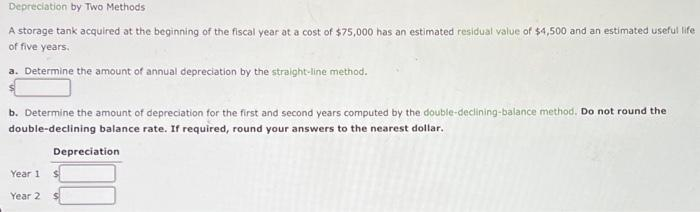Home / Expert Answers / Accounting / a-storage-tank-acquired-at-the-beginning-of-the-fiscal-year-at-a-cost-of-75-000-has-an-estimated-r-pa724

# (Solved): A storage tank acquired at the beginning of the fiscal year at a cost of \$75,000 has an estimated r ...A storage tank acquired at the beginning of the fiscal year at a cost of has an estimated residual value of and an estimated useful life of five years. a. Determine the amount of annual depreciation by the straight-line method. b. Determine the amount of depreciation for the first and second years computed by the double-declining-balance method, Do not round the double-declining balance rate. If required, round your answers to the nearest dollar.

We have an Answer from Expert

Depreciation expense is the amount that reduces the value of the asset. The reduction is usually a non-cash expense and it is recorded as the asset keeps on degrading as we keep on using them.

There are various methods of depreciation. The methods used in the solutions are
Straight line method: The straight line depreciates the asset equally over the useful life of the asset. The depreciation is distributed equally over the useful life.
Double-declining balance method: This method depreciates assets at double the rate of the straight-line method.
The straight-line depreciation expense is calculated as the cost of the asset minus the salvage value divided by the useful life of the asset.

Double declining balance depreciation expense is calculated as the book value of the asset at the beginning of the year multiplied by the depreciation rate.
We have an Answer from Expert